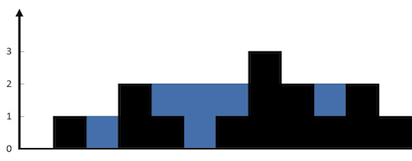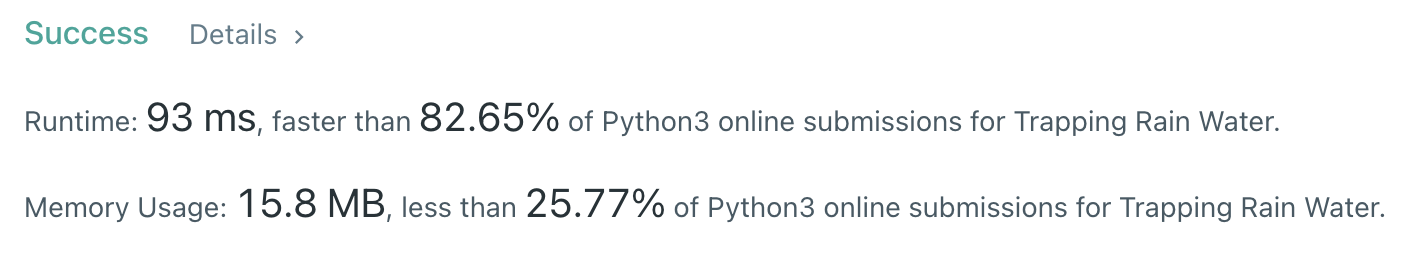Algorithm Puzzles everyday every week sometimes: Trapping Rain Water

## Puzzle

Puzzle from leetcode:

Given n non-negative integers representing an elevation map where the width of each bar is 1, compute how much water it can trap after raining.

Example 1:## Solution

### Two point with bar

My first solution is using two point with a bar:

But this solution will have time complexity up to `O(n*max(height))`, which makes it failed at some test cases on leetcode due to exceed time limit.

### Optimized two point

This optimized solution will try to find the two max height blocks:

In this case time complexity is `O(n)`: